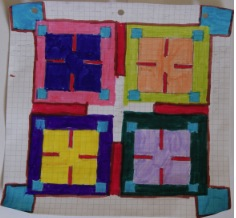You may also likeExploring Wild & Wonderful Number Patterns

EWWNP means Exploring Wild and Wonderful Number Patterns Created by Yourself! Investigate what happens if we create number patterns using some simple rules.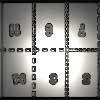Magazines

Let's suppose that you are going to have a magazine which has 16 pages of A5 size. Can you find some different ways to make these pages? Investigate the pattern for each if you number the pages.Pebbles

Place four pebbles on the sand in the form of a square. Keep adding as few pebbles as necessary to double the area. How many extra pebbles are added each time?

The Numbers Give the Design

Age 7 to 11 Challenge Level:

We had a collection of responses from Hymers College School. Katie wrote that while doing the challenge she found out a lot of different shapes the different tables made. She added that some people may say "How would you know when to end the sequence?". But the answer was that the shapes will end where you started. On a few of the tables she figured that once you have got the hang of the pattern you would be able to do the sequence backwards like on the $2$ times table. Ishita wrote:-

The things I have discovered are in the $7$ times table, in which I experimented on and I found out that in the $7$ times table, if on Microsoft Windows Logo, if you turn left $45$ degrees, it takes $8$ times before it meets with the point were it started and the angles are a lot larger, it is actually $2$ times as large as the $7$ times table in $90$ degrees.

When I do the $7$ times table, but then I turn left $90$ degrees it is a totally different shape and it only takes $4$ times to connect to the start from the end, and in comparison, it is a much smaller shape. This means that if I have a different angle on one of the times tables, for example the $3$s, it would be different in comparison of size, quantity in times of having it meet the start and in the style of the shape.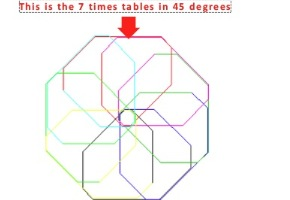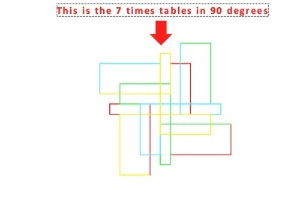From the same school we also had responses from Jessica, Jamie and Quintin. Here are their pictures:-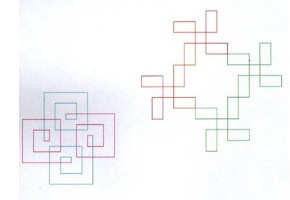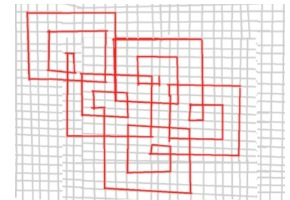A teacher from Stanmore Primary School wrote:-

Key Stage 1 maths group have investigated what happens if they use the three times table to draw their pattern. They found that the pattern repeated and that it rotated. The tricky bit was keeping track of which way to turn and making sure that you drew a straight line!

Next week they are going to investigate what happens if they use a different times table to create their pattern. They made some predictions: 'it might be the same pattern but a different size' and 'it might be a different pattern but it will still repeat and still rotate'.

They thought that it was amazing that using numbers could create a repeating pattern.

Here are some images they sent in: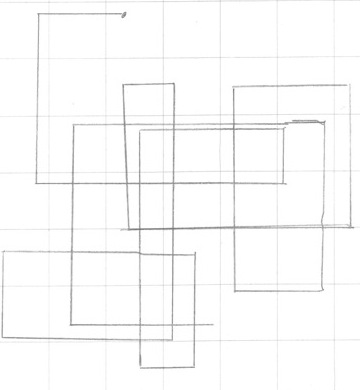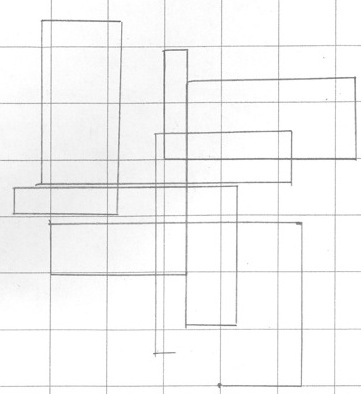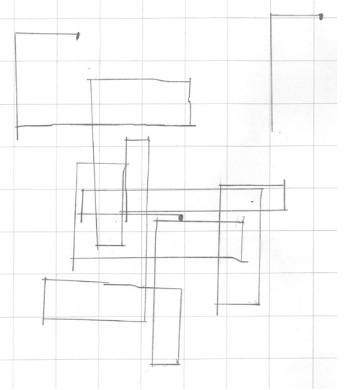Nina from Wolfson Hillel School sent us the following notes. These impressed me; particularly her ideas which led to the picture.

For my design I chose to use prime numbers. I made a pattern which went $3,2,7,5,1,5,7,2,3$; once I had gone round four times and had made a full shape I decided to make smaller version of it inside it. I knew that for my full shape I needed a square that measured $21$cms by $21$cms so I measured inside the shape and I found I could make four squares that measured $7$cms by $7$cms so I divided all the numbers by three as $21$ divided by $3$ equals $7$. Then I coloured all the bits in between $5,1,5$ red, all the corners blue and the rest I just chose nice colours, then I went over the regular sized shape's outline in brown.